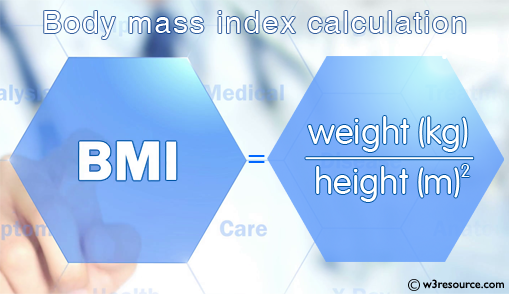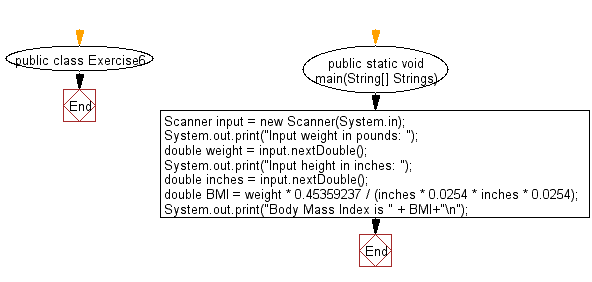﻿ Java exercises: Compute body mass index (BMI) - w3resource# Java Data Type Exercises: Compute body mass index (BMI)

## Java Data Type: Exercise-6 with Solution

Write a Java program to compute body mass index (BMI).

BMI: The BMI is defined as the body mass divided by the square of the body height, and is universally expressed in units of kg/m2, resulting from mass in kilograms and height in metres.

Test Data
Input weight in pounds: 452
Input height in inches: 72Sample Solution:

Java Code:

``````import java.util.Scanner;
public class Exercise6 {

public static void main(String[] Strings) {

Scanner input = new Scanner(System.in);

System.out.print("Input weight in pounds: ");
double weight = input.nextDouble();

System.out.print("Input height in inches: ");
double inches = input.nextDouble();

double BMI = weight * 0.45359237 / (inches * 0.0254 * inches * 0.0254);
System.out.print("Body Mass Index is " + BMI+"\n");
}
}
```
```

Sample Output:

```Input weight in pounds: 452
Input height in inches: 72
Body Mass Index is 61.30159143458721
```

Flowchart:Java Code Editor:

Improve this sample solution and post your code through Disqus

What is the difficulty level of this exercise?

﻿

## Java: Tips of the Day

Checks if a string is upper case:

```public static boolean isUpperCase(String input) {
return Objects.equals(input, input.toUpperCase());
}
```

Ref: https://bit.ly/39Hpo84